Next: Isentropic Flow Up: One-Dimensional Compressible Inviscid Flow Previous: Introduction

# Thermodynamic Considerations

Consider a quantity of gas contained in some form of enclosure. If the system is left alone for a sufficiently long time then it will attain an equilibrium state in which all macroscopically measurable quantities become independent of time. Examples of such quantities are the mass,, the pressure,, the volume,, and the absolute temperature,. It is helpful to distinguish between so-called extensive and intensive equilibrium quantities. A quantity is said to be extensive if it is proportional to the mass of the gas present in the enclosure. On the other hand, a quantity is said to be intensive if it is independent of the mass. Thus, the mass,, and volume,, are extensive quantities, whereas the pressure,, and the absolute temperature,, are intensive. For every extensive quantity (except the mass), we can introduce a corresponding intensive quantity by dividing by the mass. For instance, the intensive quantity that corresponds to the volume,, is the specific volume,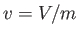, which is simply the volume per unit mass (i.e., the volume of a unit mass of gas). Of course,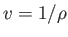, whereis the mass density.

A gas is said to be ideal if it obeys the law (Reif 1965),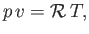(14.1)

where the constant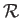is termed the specific gas constant. This quantity is related to the molar gas constant,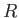, introduced in Section 1.15, according to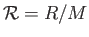, where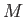is the molar mass--that is, the mass of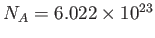gas molecules. Here,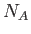is Avogadro's number (Reif 1965).

For all gases, whether in mean motion, or not, there exists an internal energy function,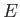, that is independent of the mean motion, and dependent only on the variables of state,,, and. Moreover, this function is such that when a small quantity of heat,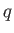, is added to the system (Reif 1965),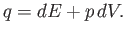(14.2)

Thus, the quantity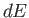is the excess of the energy supplied over the mechanical work done by the pressure. Of course, internal energy is an extensive quantity. The corresponding intensive quantity is the specific internal energy,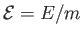. In an ideal gas, the specific internal energy,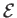, is a function of the absolute temperature,, alone (Reif 1965). It follows that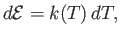(14.3)

and Equation (14.2) becomes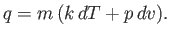(14.4)

The heat capacity at constant volume of the gas is defined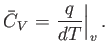(14.5)

Thus, Equation (14.4) implies that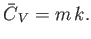(14.6)

It is clear that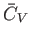is an extensive quantity. The corresponding intensive quantity is the specific heat capacity at constant volume,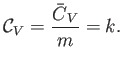(14.7)

Finally, the molar specific heat capacity at constant volume, which is the heat capacity of Avogadro's number of gas molecules, takes the form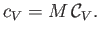(14.8)

It follows, from the preceding equations, that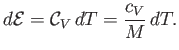(14.9)

In an ideal gas,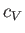is a constant, independent of the temperature (Reif 1965), in which case the previous equation can be integrated to give [cf., Equation (1.83)]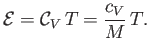(14.10)

Equation (14.1) yields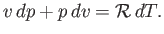(14.11)

Combining the previous equation with Equations (14.4) and (14.7), we obtain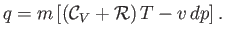(14.12)

Now, the heat capacity at constant pressure of the gas is defined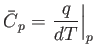(14.13)

Thus, Equation (14.12) implies that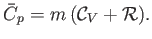(14.14)

It is clear that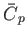is an extensive quantity. The corresponding intensive quantity is the specific heat capacity at constant pressure,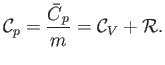(14.15)

Finally, the molar specific heat capacity at constant pressure, which is the heat capacity of Avogadro's number of gas molecules, takes the form [cf., Equation (1.86)]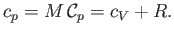(14.16)

The molar specific heat capacity at constant pressure of an ideal gas is a constant, independent of the temperature.

The enthalpy of a gas is defined (Reif 1965)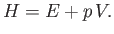(14.17)

This quantity is obviously extensive. The corresponding intensive quantity is the specific enthalpy,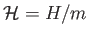. It follows that, for an ideal gas,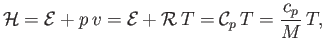(14.18)

where use has been made of Equations (14.1), (14.10), (14.15), and (14.16).

In the preceding analysis, we denoted a small quantity of heat by, rather than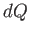, because there is, in general, no function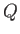of whichis an exact differential. However, we can write (Reif 1965)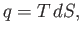(14.19)

whereis the differential of an extensive function,, called the entropy. To justify the previous expression, note from Equations (14.1), (14.4) and (14.7) that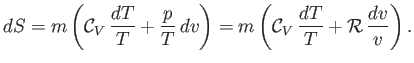(14.20)

However, Equation (14.1) yields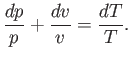(14.21)

Hence, we obtain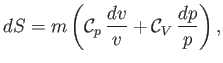(14.22)

where use has been made of Equation (14.15). In an ideal gas,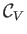and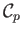are constants, independent of, so the previous expression can be integrated to give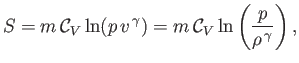(14.23)

where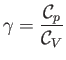(14.24)

is the constant ratio of specific heats. Obviously, the specific entropy is defined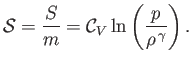(14.25)

Furthermore, it follows from Equations (14.2), (14.17), and (14.19) that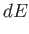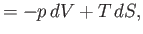(14.26)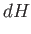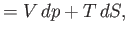(14.27)

or, equivalently,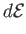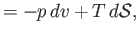(14.28)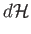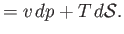(14.29)Next: Isentropic Flow Up: One-Dimensional Compressible Inviscid Flow Previous: Introduction
Richard Fitzpatrick 2016-03-31